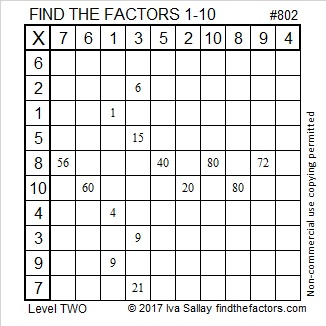# 1759 Pie Over Two

### Today’s Puzzle:

Today in the United States many students will celebrate pi day by eating pie. Today’s puzzle looks a little like a pie that has been cut in half, so I’m calling it pie over two, abbreviated as “π/2”.

Write the numbers 1 to 12 in the first column and again in the top row so that those numbers and the given clues make a multiplication table. Be sure to use logic every step of the way.### Factors of 1759:

• 1759 is a prime number.
• Prime factorization: 1759 is prime.
• 1759 has no exponents greater than 1 in its prime factorization, so √1759 cannot be simplified.
• The exponent in the prime factorization is 1. Adding one to that exponent we get (1 + 1) = 2. Therefore 1759 has exactly 2 factors.
• The factors of 1759 are outlined with their factor pair partners in the graphic below.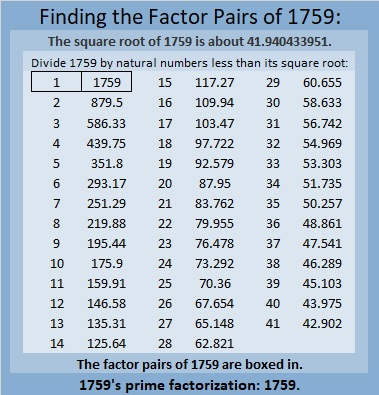How do we know that 1759 is a prime number? If 1759 were not a prime number, then it would be divisible by at least one prime number less than or equal to √1759. Since 1759 cannot be divided evenly by 2, 3, 5, 7, 11, 13, 17, 19, 23, 29, 31, 37, or 41, we know that 1759 is a prime number.

### More About the Number 1759:

Like every other odd number, 1759 is the difference of two squares:
880² – 879² = 1759.

OEIS.org informs us that 1759 is only the 17th Eisenstein-Mersenne prime number.

# 1613 Comparing π and √10

### Venn Diagram Pies:

In the United States many people celebrate pi day. This year it will be one hour shorter as we move to Daylight Saving Time. Since it will be on a Sunday, it might not get as much attention in school. Do we make too much of a deal about the number pi? It’s about 0.02 less than √10, an important, yet less-known number. I compare the two numbers in this Venn diagram: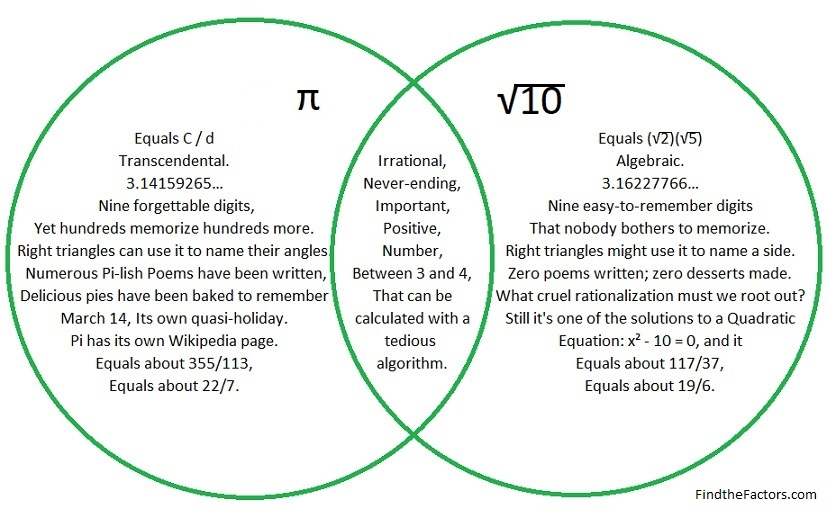We ought to take advantage of any reason to celebrate anything and everything in mathematics. I will be making some kind of pie to celebrate pi day, and I hope you do the same,

Now let’s move on to the ….

### Factors of 1613:

• 1613 is a prime number.
• Prime factorization: 1613 is prime.
• 1613 has no exponents greater than 1 in its prime factorization, so √1613 cannot be simplified.
• The exponent in the prime factorization is 1. Adding one to that exponent we get (1 + 1) = 2. Therefore 1613 has exactly 2 factors.
• The factors of 1613 are outlined with their factor pair partners in the graphic below.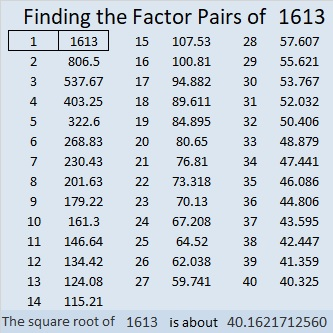How do we know that 1613 is a prime number? If 1613 were not a prime number, then it would be divisible by at least one prime number less than or equal to √1613. Since 1613 cannot be divided evenly by 2, 3, 5, 7, 11, 13, 17, 19, 23, 29, 31, or 37, we know that 1613 is a prime number.

### More about the Number 1613:

1613 is the sum of two squares:
38² + 13² = 1613.

1613 is the hypotenuse of a Pythagorean triple:
988-1275-1613, calculated from 2(38)(13), 38² – 13², 38² + 13².

Here’s another way we know that 1613 is a prime number: Since its last two digits divided by 4 leave a remainder of 1, and 38² + 13² = 1613 with 38 and 13 having no common prime factors, 1613 will be prime unless it is divisible by a prime number Pythagorean triple hypotenuse less than or equal to √1613. Since 1613 is not divisible by 5, 13, 17, 29, or 37, we know that 1613 is a prime number.

# 802 Pi Day at Smith’s

In the United States tomorrow’s date is written 3-14. Because 3.14 is a famous approximation for π (pi), people all over the country will eat pie to celebrate Pi Day. This afternoon I took a picture of this sign and the pie display at my local Smith’s Food and Drug.I took that picture right when I walked into the store, but there were no pies on display for National Pi Day.About 15 minutes later I returned to the display to take another picture. Now there were pies on the table! I told a salesperson who I think worked on the display that I was going to take a picture and put it on my blog. She asked what kind of a blog I wrote. I told her a math blog. She looked puzzled and asked why I would want to put a picture of pies on a math blog. Then she turned around, looked at the display, and said something like, “Oh, now I get it, the number pi.”

How do you choose between apple, cherry, or peach pie? It’s much easier if you choose two and then you can get a free 8 oz. Cool Whip, too. Yummy.

If by chance you prefer pizza pi, here’s a thought from twitter that is often repeated in March:

And here’s some original artwork that displays pi in a way I had never thought of before:

BREAKING: secret of Pi revealed #PiDay pic.twitter.com/Ao8BQp31jd

You can also look here for a million digits of pi.

But pi is not the only interesting number in the world. Every number has its own curiosities. Let me tell you some reasons to get excited about the number 802:

802 is the sum of two squares:

• 21² + 19² = 802

So 802 is the hypotenuse of a Pythagorean triple:

• 80-798-802, which is 2 times another triple: 40-399-401.

It also means something else: Since odd numbers 21 and 19 have no common prime factors, 802 can be evenly divide by 2. Duh. . ., but it also means that unless 802 is also divisible by 5, 13, or 17, its only factors will be 2 and a prime number! Why are those three numbers the only ones I care about? Because they are the only prime number Pythagorean triple hypotenuses less than √802 ≈ 28.3.

Guess what? 5, 13, and 17 do not divide evenly into 802, so 802 is the product of 2 and a prime number which happens to be 401.

• 802 is a composite number.
• Prime factorization: 802 = 2 x 401
• The exponents in the prime factorization are 1 and 1. Adding one to each exponent and multiplying we get (1 + 1)(1 + 1) = 2 x 2 = 4. Therefore 802 has exactly 4 factors.
• Factors of 802: 1, 2, 401, 802
• Factor pairs: 802 = 1 x 802 or 2 x 401
• 802 has no square factors that allow its square root to be simplified. √802 ≈ 28.3196045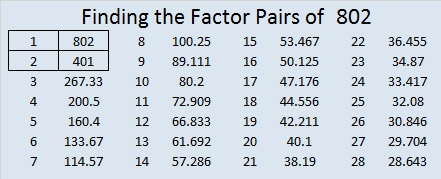Today’s puzzle is number 802 to distinguish it from every other puzzle I’ve made. Writing the numbers 1 – 10 in both the top row and the first column so that the factors and the clues work together as a multiplication table is as easy as pie!Print the puzzles or type the solution on this excel file: 10-factors 801-806

And here is a little more about the number 802:

802 is the sum of 8 consecutive prime numbers:

• 83 + 89 + 97 + 101 + 103 + 107 + 109 + 113 = 802

802 can also be written as the sum of three squares three different ways:

• 28² + 3² + 3² = 802
• 27² + 8² + 3² = 802
• 24² + 15² + 1² = 802

802 is also a palindrome in two other bases:

• 414 BASE 14 because 4(196) + 1(14) + 4(1) = 802
• 202 BASE 20 because 2(400) + 0(20) + 2(1) = 802